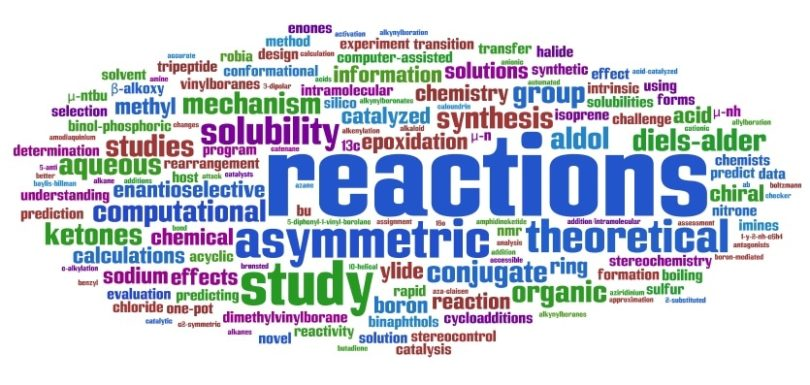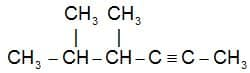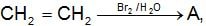Engineering Entrance Sample Papers

MU OET Chemistry Sample PaperMET (Manipal Chemistry) Chemistry Practice Questions:

Ques: In which bond angle is the highest
(a) sp3
(b) sp2
(c) sp
(d) sd3d
Ans. (c)

Ques: Which of the following species has the highest ionisation potential
(a) Li+
(b) Mg+
(c) Al+
(d) Ne
Ans. (d)

Related: AEEE Maths Question Paper

Ques: In which of the following metal carbonate which metal carbonate is decomposed on heating
(a) MgCO3
(b) Na2CO3
(c) K2CO3
(d) Pb2CO3
Ans. (a)

Ques: The catalyst used in the manufacture of methanol from water gas is
(a) V2O5
(b) Ni + Mo
(c) ZnO + Cr2O3
(d) Pt + W
Ans. (c)

Ques: Commercial detergents mainly contain
(a) RCOONa
(b) RONa
(c) RSNa
(d) ROSO2Na
Ans. (a)

Related: CBSE Physics Question Paper

Ques: In which of the following processes shape-selective catalysis is occurring
(a) Conversion of alcohol to gasoline
(b) Synthesis of methanol from CO and H2
(c) Polymerisation of ethylene
(d) Synthesis of ammonia
Ans. (a), (c)

Ques: A white sodium salt dissolves readily in water to give a solution which is neutral to litmus. When silver nitrate solution is added to the solution, a white precipitate is formed which does not dissolve in dilute nitric acid. The anion could be
(a) SO42–
(b) CO32–
(c) S2–
(d) Cl
Ans. (d)

Ques: The colour of CuCr2O7 solution in water is green because
(a) Cr2O72– ions are green
(b) Cu++ ions are green
(c) Both ions are green
(d) Cu++ ions are blue and Cr2O72– ions are yellow
Ans. (d)

Ques: The poisonous gas that comes out with petrol burning in a car is
(a) CH4
(b) C2H6
(c) CO2
(d) CO
Ans. (d)

Related: BITSAT Physics Question Bank

Ques: Neutral ferric chloride is added to the aqueous solution of acetate. The blood red colour is obtained, it is due to the compound
(a) Fe(OH)2
(b) Fe(OH)3
(c) Fe(CH3COO)3
(d) Fe(OH)2(CH3COO)
Ans. (c)

Ques: A salt solution is treated with chloroform drops. Then it is shaked with chlorine water. Chloroform layer becomes violet. Solution contains
(a) NO2 ion
(b) NO3 ion
(c) Br ion
(d) I ion
Ans. (d)

Ques: Indicator type silica gel used as a dehumidifier contains
(a) Cu2+ ions
(b) Ni2+ ions
(c) Co2+ ions
(d) Fe2+ ions
Ans. (c)

Ques: The radium and uranium atoms in a sample of uranium mineral are in the ratio of 1 : 28 x 106. If half-life period of radium is 1620 years, the half-life period of uranium will be
(a) 45.3 x 109 years
(b) 45.3 x 1010 years
(c) 4.53 x 109 years
(d) 4.53 x 1010  years
Ans. (c)

Ques: The order of dipole moments of the following molecules is
(a) CHCl3 > CH2Cl2 > CH3Cl > CCl4
(b) CH2Cl2 > CH3Cl > CHCl3 > CCl4
(c) CH3Cl > CH2Cl2 > CHCl3 > CCl4
(d) CH2Cl2 > CHCl3 > CH3Cl > CCl4
Ans. (d)

Ques: In which of the following, the reaction proceeds towards completion
(a) K = 103
(b) K = 10–2
(c) K = 10
(d) K = 1
Ans. (a)

Ques: Which one of the following non-ideal solutions shows the negative deviation
(a) CH3COCH3 + CS2
(b) C6H6 + CH3COCH­3
(c) CCl4 + CHCl3
(d) CH3COCH3 + CHCl3
Ans. (d)

Ques: C2H5I and Ag2O reacts to produce
(a) C2H6
(b) C2H5 – C2H5
(c) C2H5 – O – C2H5
(d) C2H5 – CH3
Ans. (c)

Related: EAMCET Chemistry Question Bank

Ques: In hydrolysis of aniline, the reagent used is
(a) Dil. HCl
(b) Acetyl chloride
(c) CH3OH
(d) None of these
Ans: (a)

Ques: The mass of neutron is nearly
(a) 10–23 kg
(b) 10–24
(c) 10–26 kg
(d) 10–27 kg
Ans: (d)

Ques: Which of the following statements (s) is (are) correct
(a) The electronic configuration of Cr is [Ar]3d54s1 (Atomic no. of Cr = 24)
(b) The magnetic quantum number may have a negative value
(c) In silver atom, 23 electrons have a spin of one type and 24 of the opposite type (Atomic no. of Ag = 47)
(d) The oxidation state of nitrogen in HN3 is –3
Ans: (a, b, c)

Ques: When N2 goes to N2+ the N – N bond distance ….. and when O2 goes to O2+, the O – O bond distance …
(a) Decrease, increase
(b) Increase, decrease
(c) Increase, increase
(d) None of these
Ans: (b)

Ques: If the coordination of Ca2+ in CaF2 is 8, then the coordination number of Fion would be
(a) 3
(b) 4
(c) 6
(d) 8
Ans: (b)

Ques: Ethylamine can be obtained by the
(a) Action of NH3 on ethyl iodide
(b) Action of NH3 on ethyl alcohol
(c) Both (a) and (b)
(d) None of the above
Ans: (c)

Ques: In which of the following solvents will AgBr have the highest solubility
(a) 10–3 M NaBr
(b) 10–3 M NH4OH
(c) Pure water
(d) 10–3 M HBr
Ans. (b)

Ques: The dissociation constant of a weak acid is  the equilibrium constant for the reaction with strong base is
(a) 1.0 x 10–5
(b) 1.0 x 10–9
(c) 1.0 x 109
(d) 1.0 x 1014
Ans: (c)

Ques: A reaction rate constant is given by k = 1.2 x 1014 e–(25000 / RT) sec–1
It means
(a) log k versus log T will give a straight line with slope as – 25000
(b) log k versus T will give a straight line with slope as – 25000
(c) log k versus log 1/T will give a straight line with slope as – 25000
(d) log k versus 1/T will give a straight line
Ans: (d)

Ques: An electric current is passed through an aqueous solution of the following. Which one shall decompose
(a) Urea
(b) Glucose
(c) AgNO3
(d) Ethyl alcohol
Ans: (c)

Ques: Mark the wrong statement
(a) Wrought iron is prepared by heating cast iron in a reverberatory furnace
(b) The impurities present in cast iron are oxidised by air
(c) The impurities are oxidised by Fe2O3
(d) CO burns with blue flame and the Si, Mn and other impurities form slag with silica
Ans. (b)

Ques: Which of the following is the most stable
(a) Pb2+
(b) Ge2+
(c) Si2+
(d) Sn2+
Ans. (a)

Ques: The solubility product constant Ksp of Mg(OH)2 is 9.0 x 10–12. If a solution is 0.010 M  with respect to Mg2+ ion, what is the maximum hydroxide ion concentration which could be present without causing the precipitation of Mg(OH)2
(a) 1.5 x 10–7 M
(b) 3.0 x 10–7 M
(c) 1.5 x 10–5 M
(d) 3.0 x 10–5 M
Ans. (d)

Ques: To an acid solution of an anion a few drops of KMnO4 solution are added. Which of the following, if present will not decolourise the KMnO4 solution
(a) NO2
(b) S2–
(c) Cl
(d) CO32–
Ans: (d)

Ques: Choose the correct IUPAC name of the compound(a) 2, 3-dimethyl-4-hexyne
(b) 4,5-dimethyl-2-hexyne
(c) 5-propyl-2-pentyne
(d) 2-propyl-3-pentyne
Ans: (b)

Ques: Which of the following is an electrophile
(a) H2O
(b) NH3
(c) AlCl3
(d) C2H5NH2
Ans. (c)

Ques: When ethyl alcohol is heated with red phosphorus and HI, then which of the following is formed
(a) C2H6
(b) CH4
(c) C3H8
(d) C2H4
Ans. (a)

Ques: In the below reaction the compound A is(a) Ethylene bromohydrin
(b) 1, 2-dibromo ethane
(c) Ethanol
(d) None of these
Ans: (a)

Ques: The oxide which is not reduced by hydrogen is
(a) Ag2O
(b) K2O
(c) Fe2O3
(d) P4O10
Ans. (b)

Ques: In which alkyl halide, SN2 mechanism is favoured maximum
(a) CH3Cl
(b) CH3CH2Cl
(c) (CH3)2CHCl
(d) (CH3)3C – Cl
Ans: (a)

Ques: The compound X on reaction with HgSO4 + H2SO4 gives Y which on oxidation gives acetic acid. X is
(a) C2H2
(b) C2H4
(c) C3H4
(d) C4H6
Ans. (a)

Ques: The ionic radii of Rb+ and Iare 1.46 Å and 2.16 Å. the most probable type of structure exhibited by it is
(a) CsCl type
(b) ZnS type
(c) NaCl type
(d) CaF2 type
Ans: (c)

Ques: The ratio of the energy of a photon of 2000 Å wavelength radiation to that of 4000 Å radiation is
(a) 1/4
(b) 4
(c) 1/2
(d) 2
Ans: (d)